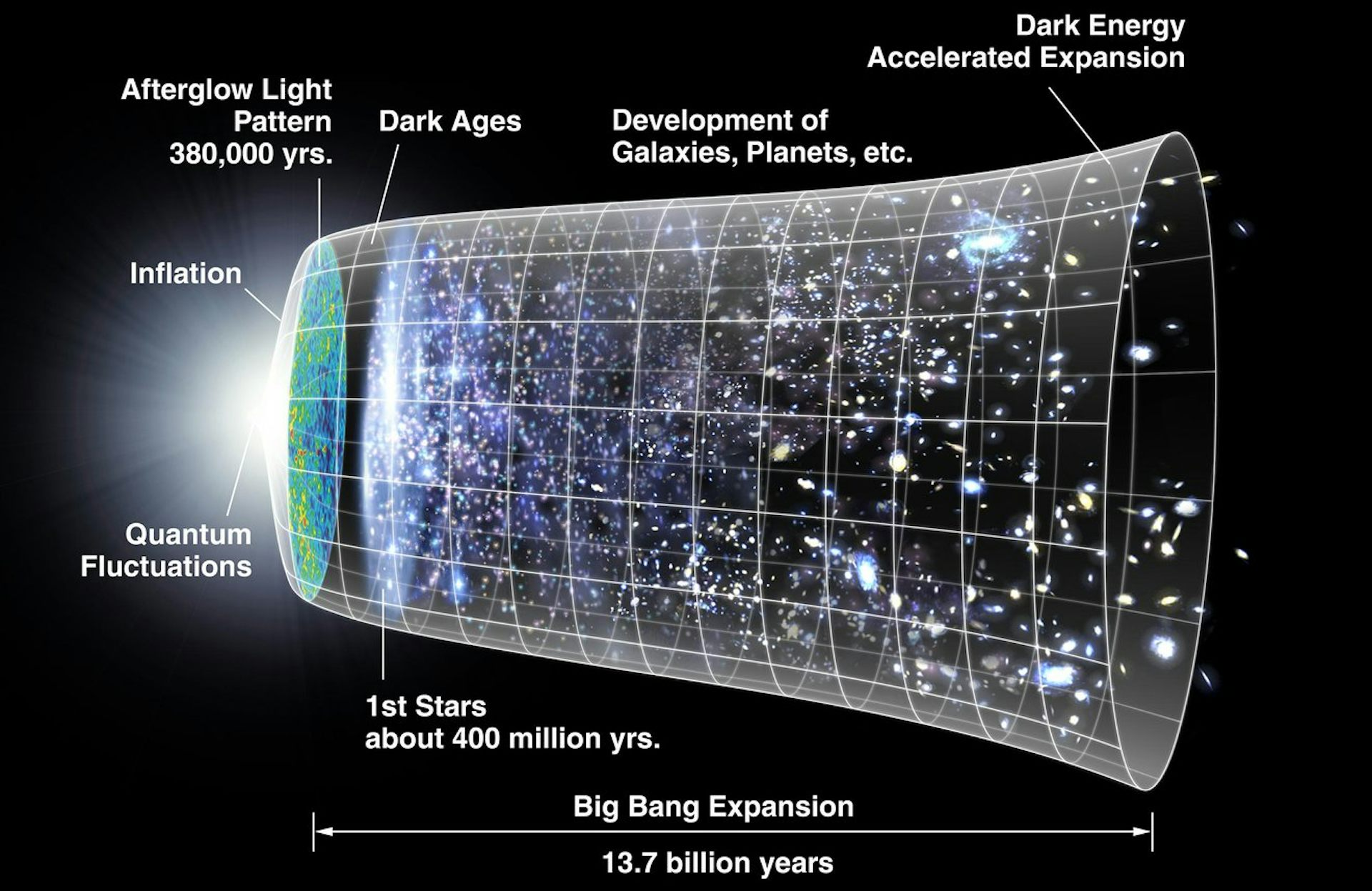# Dark stars

| November 20, 2023Astronomers have known for decades that the universe is expanding. When they use telescopes to observe faraway galaxies, they see that these galaxies are moving away from Earth.

To astronomers, the wavelength of light a galaxy emits is longer the faster the galaxy is moving away from us. The farther away the galaxy is, the more its light has shifted toward the longer wavelengths on the red side of the spectrum – so the higher the “redshift.”

Because the speed of light is finite, fast, but not infinitely fast, seeing something far away means we’re looking at the thing how it looked in the past. With distant, high-redshift galaxies, we’re seeing the galaxy when the universe was in a younger state. So “high redshift” corresponds to the early times in the universe, and “low redshift” corresponds to the late times in the universe.

But as astronomers have studied these distances, they’ve learned that the universe is not just expanding – its rate of expansion is accelerating. And that expansion rate is even faster than the leading theory predicts it should be, leaving cosmologists like me puzzled and looking for new explanations.

#### Dark energy and a cosmological constant

Scientists call the source of this acceleration dark energy. We’re not quite sure what drives dark energy or how it works, but we think its behavior could be explained by a cosmological constant, which is a property of spacetime that contributes to the expansion of the universe.

Albert Einstein originally came up with this constant – he marked it with a lambda in his theory of general relativity. With a cosmological constant, as the universe expands, the energy density of the cosmological constant stays the same.

Imagine a box full of particles. If the volume of the box increases, the density of particles would decrease as they spread out to take up all the space in the box. Now imagine the same box, but as the volume increases, the density of the particles stays the same.

It doesn’t seem intuitive, right? That the energy density of the cosmological constant does not decrease as the universe expands is, of course, very weird, but this property helps explain the accelerating universe.

#### A standard model of cosmology

Right now, the leading theory, or standard model, of cosmology is called “Lambda CDM.” Lambda denotes the cosmological constant describing dark energy, and CDM stands for cold dark matter. This model describes both the acceleration of the universe in its late stages as well as the expansion rate in its early days.

Specifically, the Lambda CDM explains observations of the cosmic microwave background, which is the afterglow of microwave radiation from when the universe was in a “hot, dense state” about 300,000 years after the Big Bang. Observations using the Planck satellite, which measures the cosmic microwave background, led scientists to create the Lambda CDM model.

Fitting the Lambda CDM model to the cosmic microwave background allows physicists to predict the value of the Hubble constant, which isn’t actually a constant but a measurement describing the universe’s current expansion rate.

But the Lambda CDM model isn’t perfect. The expansion rate scientists have calculated by measuring distances to galaxies, and the expansion rate as described in Lambda CDM using observations of the cosmic microwave background, don’t line up. Astrophysicists call that disagreement the Hubble tension.The universe is expanding faster than predicted by popular models in cosmology.

#### The Hubble tension

Over the past few years, I’ve been researching ways to explain this Hubble tension. The tension may be indicating that the Lambda CDM model is incomplete and physicists should modify their model, or it could indicate that it’s time for researchers to come up with new ideas about how the universe works. And new ideas are always the most exciting things for a physicist.

One way to explain the Hubble tension is to modify the Lambda CDM model by changing the expansion rate at low redshift, at late times in the universe. Modifying the model like this can help physicists predict what sort of physical phenomena might be causing the Hubble tension.

For instance, maybe dark energy is not a cosmological constant but instead the result of gravity working in new ways. If this is the case, dark energy would evolve as the universe expands – and the cosmic microwave background, which shows what the universe looked like only a few years after its creation, would have a different prediction for the Hubble constant.

But, my team’s latest research has found that physicists can’t explain the Hubble tension just by changing the expansion rate in the late universe – this whole class of solutions falls short.

#### Developing new models

To study what types of solutions could explain the Hubble tension, we developed statistical tools that enabled us to test the viability of the entire class of models that change the expansion rate in the late universe. These statistical tools are very flexible, and we used them to match or mimic different models that could potentially fit observations of the universe’s expansion rate and might offer a solution to the Hubble tension.

The models we tested include evolving dark energy models, where dark energy acts differently at different times in the universe. We also tested interacting dark energy-dark matter models, where dark energy interacts with dark matter, and modified gravity models, where gravity acts differently at different times in the universe.

But none of these could fully explain the Hubble tension. These results suggest that physicists should study the early universe to understand the source of the tension.